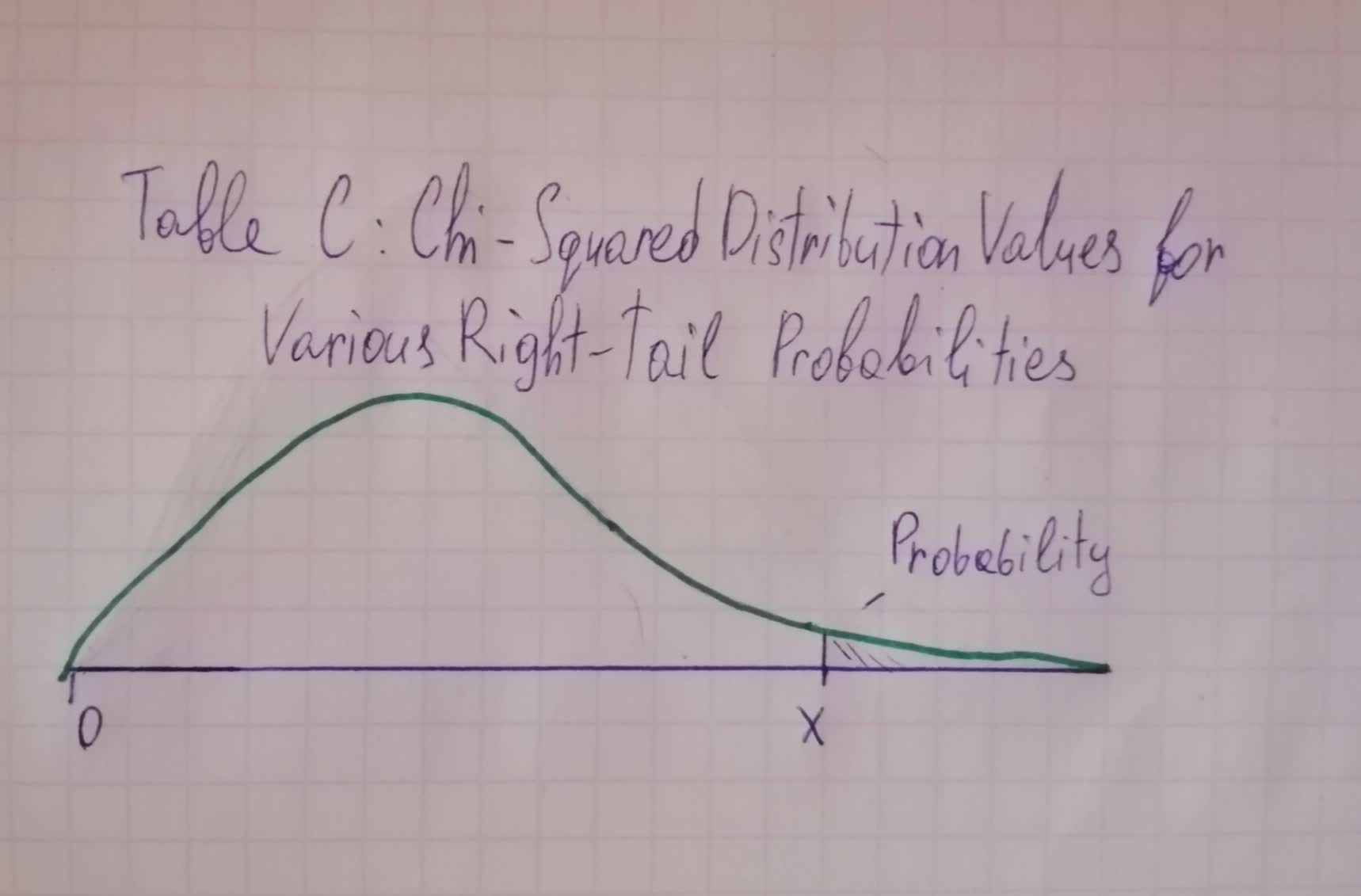# If the chi-square test statistic what is the p-value you would report? Use Table C.Remember to calculate the df first.Zoe Oneal 2021-06-11 Answered

$$\begin{array}{cc}\hline & \text{Afraid to walk at night?} \\ \hline & \text{Yes} & \text{No} & \text{Total} \\ \hline \text{Male} & 173 & 598 & 771 \\ \hline \text{Female} & 393 & 540 & 933 \\ \hline \text{Total} & 566 & 1138 & 1704 \\ \hline \text{Source:}2014\ GSS \end{array}$$
If the chi-square $$(\chi 2)$$ test statistic $$=73.7$$ what is the p-value you would report? Use Table C.Remember to calculate the df first.
$$P>0.250$$
$$P=0.01$$
$$P<0.001$$
$$P<0.002$$$$\begin{array}{|c|cc|}\hline & \text{Right-Tail Probability} \\ \hline df & 0.250 & 0.100 & 0.050 & 0.025 & 0.010 & 0.005 & 0.001 \\ \hline 1 & 1.32 & 2.71 & 3.84 & 5.02 & 6.63 & 7.88 & 10.83 \\ 2 & 2.77 & 4.61 & 5.99 & 7.38 & 9.21 & 10.60 & 13.82 \\ 3 & 4.11 & 6.25 & 7.81 & 9.35 & 11.34 & 12.84 & 16.27 \\ 4 & 5.39 & 7.78 & 9.49 & 11.14 & 13.28 & 14.86 & 18.47 \\ 5 & 6.63 & 9.24 & 11.07 & 12.83 & 15.09 & 16.75 & 20.52 \\ 6&7.84&10.64&12.59&14.45&16.81&18.55&22.46 \\ 7&9.04&12.02&14.07&16.01&18.48&20.28&24.32\\ 8&10.22&13.36&15.51&17.53&20.09&21.96&26.12 \\ 9&11.39&14.68&16.92&19.02&21.67&23.59&27.88 \\ 10&12.55&15.99&18.31&20.48&23.21&25.19&29.59 \\ 11&13.70&17.28&19.68&21.92&24.72&26.76&31.26 \\ 12&14.85&18.55&21.03&23.34&26.22&28.30&32.91 \\ 13&15.98&19.81&22.36 & 24.74 & 27.69 & 29.82 & 34.53 \\ 14 & 17.12 & 21.06 & 23.68 & 26.12 & 29.14 & 31.32 & 36.12 \\15 & 18.25 & 22.31 & 25.00 & 27.49 & 30.58 & 32.80 & 37.70 \\ 16 & 19.37 & 32.54 & 26.30 & 28.85 & 32.00 & 34.27 & 39.25 \\ 17 & 20.49 & 24.77 & 27.59 & 30.19 & 33.41 & 35.72 & 40.79 \\ 18 & 21.60 & 25.99 & 28.87 & 31.53 & 34.81 & 37.16 & 42.31 \\ 19 & 22.72 & 27.20 & 30.14 & 32.85 & 36.19 & 38.58 & 43.82 \\ 20 & 23.83 & 28.41 & 31.41 & 34.17 & 37.57 & 40.00 & 45.32 \\ \hline \end{array}$$

• Questions are typically answered in as fast as 30 minutes

### Plainmath recommends

• Get a detailed answer even on the hardest topics.
• Ask an expert for a step-by-step guidance to learn to do it yourself.saiyansruleA

Step 1
The degrey of freedom for:
Rows: $$=2=m$$
Columns: $$=2=n$$
$$df=(m-1)(n-1)$$
Step 2
$$df$$ of $$\chi^{2}=(2-1)\times(2-1)=1$$
The test statistic $$\chi^{2}=73.7$$
The P-value $$=0.0000<0.001$$